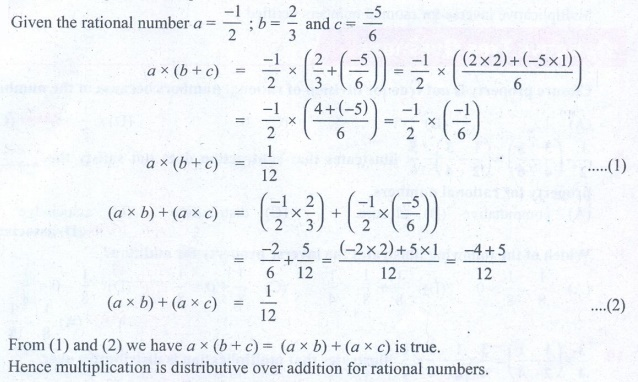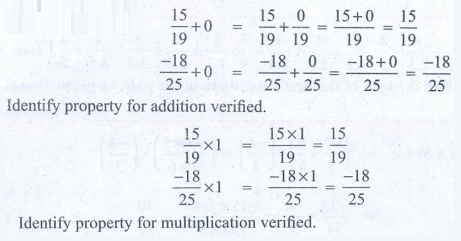Home | | Maths 8th Std | Exercise 1.3 (Properties of Rational Numbers)

# Exercise 1.3 (Properties of Rational Numbers)

8th Maths : Chapter 1 : Numbers : Properties of Rational Numbers : Exercise 1.3 : Numerical solved problems and Text book back Numerical problems Questions with Solution

Exercise 1.3

1. Verify the closure property for addition and multiplication for the rational numbers 5/7 and 8/9 .

Solution:Let a = −5/7  and b = 8/9 be the given rational numbers.

a + b = −5/7 + 8/9

= [ (−5 × 9) + (8 × 7)] / [7 × 9]

= [−45 + 56] / 63 = 11 / 63  is in Q.

i.e a + b = [−5/7] + [8/9] = 11/63  is in Q.

Closure property is true for addition of rational numbers.

Closure property for multiplication

Let  a = −5/7 and b = 8/9

a × b = −5/7 × 8/9 = −40/63 is in Q.

Closure property is true for multiplication of rational numbers.

2. Verify the commutative property for addition and multiplication for the rational numbers 10/11 and 8/33

Solution:Let a = −10/11 and b = −8/33 be the given rational numbers.

Now a + b = −10/11 + (−8/33) = [ (−10 × 3) + (−8 × 1)] / 33 = [−30 + (−8)] / 33

a + b = −38/33        ………….(1)

b + a =  (−8/33) + (−10/11) = [ (−8 × 1) + ((−10) × 3)] / 33   = [−8 + (−30)] / 33

b + a =  − 38 / 33 …………..(2)

From (1) and (2)

a + b = b + a and hence addition is commutative for rational numbers.

Further a × b = −10/11 × (−8/33) = 80/363

a × b = 80/363  …………….(3)

b × a = −8/33 × (−10/11) = 80/363

b × a = 80/363 ………….(4)

From (3) and (4) a × b = b × a

Hence multiplication is commutative for rational numbers.

3. Verify the associative property for addition and multiplication for the rational numbers 7/9 , 5/6 and 4/3.

Solution:Let a = −7/9, b = 5/6,  c = −4/3 be the given rational numbers.

(a + b) + c = (−7/9 + 5/6) + (−4/3) = ( [−7 × 2 + 5 × 3] / 18 ) + (−4/3)

= ( [−14 + 15] / 18) + (−4/3) = 1/18 + (−4/3)

= [1 + (−4) × 6] / 18 = [ 1 + (−24) ] / 18 = −23/18 ……..(1)

a + (b + c ) = −7/9 + (5/6 + (−4)/3) = −7/9 + ( [5 + (−4) 2] / 6)

= −7/9 + ( [5 + (−8)] / 6) = −7/9 + (−3/6) = −7/9 + (−1/2)

= [−7 × 2 + (−1) × 9] / 18 = [ −14 + (−9) ] / 18 = −23/18 ……..(2)

From (1) and (2), (a + b) + c = a + (b + c) is true for rational numbers.

Now

(a × b) × c = (−7/9 × 5/6) × (−4/3) = ( [−7 × 5] / [9 × 6] ) × (−4/3)

= −35/54 × −4/3 = [−35 × (−4)] / [54 × 3] = 70/81 ………(1)

a × (b x c) = −7/9 × ([5/6] × [−4/3]) = −7/9 ×  [5 × (−2)] / [3 × 3]

= −7/9 × (−10)/9  = 70/81 ………(2)

From (1) and (2) (a × b) × c = a × (b × c) is true for addition and multiplication for the rational numbers.

Thus associative property.

4. Verify the distributive property a × (b + c ) = ( a × b ) + ( a + c) for the rational numbers a = -1/2 , b = 2/3 and c = -5/6 .

Solution:Given the rational number a = −1/2 ; b = 2/3 and c = −5/6

a × (b + c) = −1/2 × (2/3 + (−5/6)) = −1/2 × ( [(2 × 2) + (−5 × 1)] /6 )

= −1/2 × ( [4 + (−5)] / 6 ) = −1/2 × (−1/6)

a × (b + c) = 1/12 ………(1)

(a × b) + (a × c) = (−1/2 × 2/3) + ( −1/2 × (−5/6) )

= −2/6 + 5/12 = [(−2 × 2) + 5 × 1] / 12 = [−4 + 5] / 12

(a × b) + (a × c) = 1/12   ………(2)

From (1) and (2) we have a × (b + c)  = (a × b) + (a × c) is true.

Hence multiplication is distributive over addition for rational numbers.

5. Verify the identity property for addition and multiplication for the rational numbers 15/19 and 18/25.

Solution:[15/19] + 0 = [15/19] + [0/19] = [15 + 0] / 19 = 15/19

[−18/25] + 0 = [−18/25] + [0/25] = [−18 + 0] / 25 = −18/25

[15/19] × 1 = [15 × 1] / 19 = 15/19

[−18/25] × 1 = [−18 × 1] / 25 = −18/25

Identify property for multiplication verified.

6. Verify the additive and multiplicative inverse property for the rational numbers 7/17 and 17/27.

Solution:−7/17 + 7/17 = [−7 + 7] / 17 = 0/17 = 0

17/27 + (−17/27) = [17 + (−17)] / 27 = 0/27 = 0

Additive inverse for rational numbers verified.

−7/17 × 17/−7 = [−7 × 17] / [17 × (−7)] = 1

17/27 × 27/17 = [17 × 27] / [27 × 17] = 1

Multiplicative inverse for rational numbers verified.

Objective Type Questions

7. Closure property is not true for division of rational numbers because of the number

(A) 1

(B) –1

(C) 0

(D) 1/2

8.illustrates that subtraction does not satisfy the ________ property for rational numbers.

(A) commutative

(B) closure

(C) distributive

(D) associative

9. Which of the following illustrates the inverse property for addition?[Answer: (A) 1/8 – 1/8 = 0]

10.illustrates that multiplication is distributive over

(B) subtraction

(C) multiplication

(D) division

We know that different operations with the same pair of rational numbers usually give different answers. Check the following calculations which are some interesting exceptions in rational numbers.

(i) 13/4 + 13/9 = 13/4 × 13/9

(ii) 169/30 + 13/15 = 169/30 ÷ 13/15

Amazing …! Isn’t it? Try a few more like these, if possible.

Think

Observe that,Use your reasoning skills, to find the sum of the first 7 numbers in the pattern given above.

Solution:

1/1.2 + 1/2.3 + 1/3.4 + 1/4.5 + 1/5.6 + 1/ 6.7 + 1/7.8 = 7 / 8

Exercise 1.3

Verify yourself for Qns 1 to 6.

7. (C) 0

8. (D) associative

9. (A) 1/8 – 1/8 = 0

10. (B) subtraction

Tags : Questions with Answers, Solution | Numbers | Chapter 1 | 8th Maths , 8th Maths : Chapter 1 : Numbers
Study Material, Lecturing Notes, Assignment, Reference, Wiki description explanation, brief detail
8th Maths : Chapter 1 : Numbers : Exercise 1.3 (Properties of Rational Numbers) | Questions with Answers, Solution | Numbers | Chapter 1 | 8th Maths